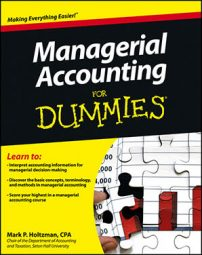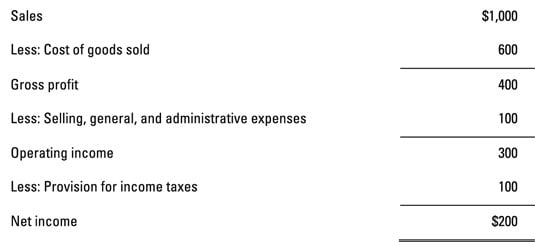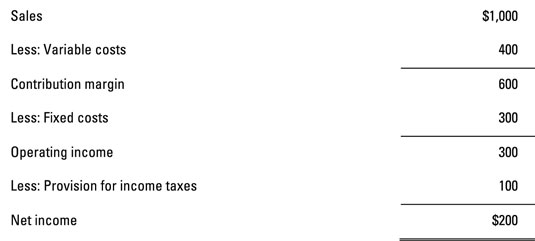##### Managerial Accounting For DummiesContribution margin measures how sales affects net income or profits. To compute contribution margin, subtract variable costs of a sale from the amount of the sale itself:

Contribution margin = Sales – Variable costs

For example, if you sell a gadget for \$10 and its variable cost is \$6, the contribution margin for the sale would be \$4 (\$10 – \$6 = \$4). Selling this gadget would increase your profit by \$4.

When computing contribution margin, subtract all variable costs, including variable manufacturing costs and variable selling, general, and administrative costs. Don’t subtract any fixed costs. You compute gross profit by subtracting cost of goods sold from sales. Because cost of goods sold usually includes a mixture of fixed and variable costs, gross profit doesn’t equal contribution margin.

You can calculate contribution margin in three forms:

• In total

• Per unit

• As a ratio

Contribution margin, in any of its forms, explains how different factors in the company — sales price, sales volume, variable costs, and fixed costs — interact. This understanding helps you make better decisions when planning sales and costs.

## Figure total contribution margin

Total contribution margin measures the amount of contribution margin earned by the company as a whole. You calculate it by using this formula:

Total contribution margin = Total sales – Total variable costs

To determine overall profitability, compare total contribution margin to fixed costs. Net income equals the excess of contribution margin over fixed costs.

You can use total contribution margin to create something called a contribution margin income statement. This document is different from a multi-step income statement, where you first subtract cost of goods sold from sales and then subtract selling, general, and administrative costs.A contribution margin income statement first subtracts the variable costs and then subtracts fixed costs. Here, variable costs include variable costs of both manufacturing and selling. Likewise, fixed costs include more manufacturing and selling costs.The contribution margin income statement makes understanding cost behavior and how sales will affect profitability easier. In Figure 9-2, the company earned \$1,000 in sales, \$400 of which went toward variable costs. This scenario resulted in \$600 of contribution margin.

These amounts — sales, variable costs, and contribution margin — change in proportion to each other. If sales were to increase by 10 percent, then variable costs and contribution margin would also increase by 10 percent; \$1,100 in sales would increase variable costs to \$440 and contribution margin to \$660.

On the other hand, fixed costs always remain the same: The \$300 in fixed costs will be \$300 regardless of any increase or decrease in sales and contribution margin.

The contribution margin income statement presents the same net income figure as a traditional income statement. However, the contribution margin income statement is not in accordance with Generally Accepted Accounting Principles (GAAP), the set of rules companies must use for external reporting. Managers can internally use a contribution margin income statement to better understand their own companies’ operations.

## Calculate contribution margin per unit

Contribution margin per unit measures how the sale of one additional unit would affect net income. You calculate it by subtracting variable costs per unit from sales price per unit, as in this formula:

Contribution margin per unit = Sales price per unit – Variable costs per unit

Say a company sells a single gadget for \$100, and the variable cost of making the gadget is \$40. Contribution margin per unit on this gadget equals \$60 (100 – 40 = 60). Therefore, selling the gadget increases net income by \$60.

Increasing the sales price doesn’t affect variable costs because the number of units manufactured, not the sales price, is what usually drives variable manufacturing costs.

Therefore, if the gadget company raises its sales price to \$105, the variable cost of making the gadget remains at \$40, and the contribution per unit increases to \$65 per unit (\$105 – \$40 = \$65). The \$5 increase in sales price goes straight to the bottom line as net income.

## Work out contribution margin ratio

Contribution margin ratio measures the percentage of sales that would increase net income. To calculate it, divide contribution margin by sales, either in total or per unit:

Contribution margin ratio = Total contribution margin / Total sales
or
Contribution margin ratio = Contribution margin per unit / Sales price per unit

Suppose a gadget selling for \$100 per unit brings in \$40 per unit of contribution margin. Its contribution margin ratio is 40 percent:

Contribution margin ratio = 40 / 100 = 40%

To find out how sales affect net income, multiply the contribution margin ratio by the amount of sales. In this example, \$1,000 in gadget sales increases net income by \$400 (\$1,000 x 40 percent = \$400).